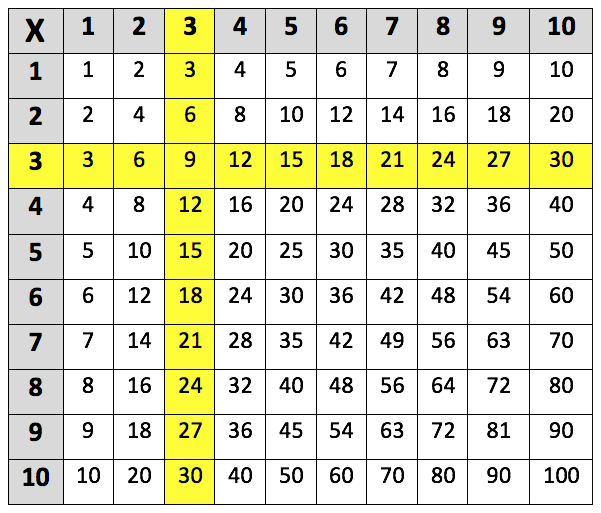Learning the 3 times table. The 3 times table up to 3 x 10 is highlighted in the multiplication grid below:This is the 3 times table up to 3 x 12:
3 x 1 = 3
3 x 2 = 6
3 x 3 = 9
3 x 4 = 12
3 x 5 = 15
3 x 6 = 18
3 x 7 = 21
3 x 8 = 24
3 x 9 = 27
3 x 10 = 30
3 x 11 = 33
3 x 12 = 36

## When do children learn the 3 times table?

In Year 2, children are taught the concept of multiplication and start by learning the 2, 5 and 10 times tables.

In Year 3, teachers move on to the 3, 4 and 8 times tables. Children should also be able to use practical and written methods of multiplying and dividing two-digit numbers. For example, 12 x 3 = 36.

By the end of Year 4, children should have been taught all the times tables up to the 12 times table. Teachers will start giving children harder multiplications to complete, these will include multiplying three-digit numbers by a one-digit number (such as 150 x 3 = 450).

Throughout Years 5 and 6, children should be confident in their times tables up to 12. They will be expected to use their knowledge of multiplication as they are taught more complex areas of maths, such as algebra.

As well as learning their times tables, children should be taught the division facts for the times tables. For the three times table, these include:

6 ÷ 3 = 2, 9 ÷ 3 = 3 and 12 ÷ 3 = 4.

## Are there any helpful tricks to learn the 3 times table?

An interesting rule to teach children with the 3 times table is that if you add up the digits of a multiple of 3, then they will always equal another multiple of 3. For example:

15    ->    1 + 5 = 6

21    ->    2 + 1 = 3

18    ->    1 + 8 = 9

93    ->    9 + 3 = 12

Whereas:

As 5 is not a multiple of 3, 32 is also not a multiple of 3.

32    ->     3 + 2 = 5

This rule can help children check their answers are correct when they have calculated a multiple of 3. It can also be useful if they are given a number and are asked if it is a multiple of 3 or not.

How to help children with their 3 times table?

There are many different methods of learning the times tables to explore, it is a good idea to try out a few of these methods and see which one seems to work best for your child.

Sometimes it is easier to learn times tables when it is made fun by playing games, as children often remember things better when they are interactive and visual.

One way to do this is by creating a times table pairs game. Cut out 24 pieces of card and write out 3 times table questions on 12 of the cards. On the other 12 cards, write out the answers and then mix up the questions and answers and lay them out on a table. Ask your child to see how quickly they can pair each question with the correct answer. If they are competitive, it can also be fun to time your child and challenge them to get quicker the next time they play the game.

## How does Learning Street help children with the 3 times table?

Repetition is key to learning your 3 times table, and in the Learning Street 8 year old courses, there is a constant focus on always practising times tables as this is the best way to improve. This is through times tables tests (to improve accuracy), tables races (to improve time), mental mathematics, maths problem solving and other fun activities such as mystery pictures.

Without a good knowledge of times tables, it can lead to problems in SATs, GCSEs and A Levels.

## Our Courses

Click through to review the courses we have available

##### Get Started

Learning Street structured courses make home study easier and more successful. There's no need to wonder which books to buy or what to do next. Everything is planned for you.

• Proven to deliver excellent results
• Used by families, private tutors and schools
• Fully planned and structured